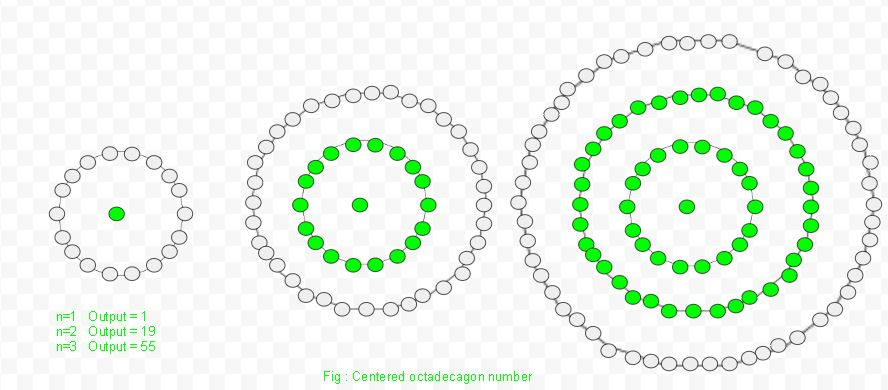Given a number n, find the nth Centered Octadecagonal number.

The Centered Octadecagonal Number represents a dot in the centre and others dot are arranged around it in successive layers of octadecagon(18 sided polygon).Examples :

```Input :  2
Output : 19

Input : 6
Output : 271
```

## Recommended: Please try your approach on {IDE} first, before moving on to the solution.

In mathematics, Centered Octadecagonal number for n-th term is given by:

``````

Below is the basic implementation of the above idea:

## C++

 `// C++ Program to find the ` `// nth centered octadecagonal ` `// number ` `#include ` `using` `namespace` `std; ` ` `  `// centered octadecagon  function ` `int` `center_octadecagon_num(``long` `int` `n) ` `{ ` `    ``// Formula to calculate nth ` `    ``// centered octadecagonal number ` `    ``return` `9 * n * n - 9 * n + 1; ` `} ` ` `  `// Driver Code ` `int` `main() ` `{ ` `    ``long` `int` `n = 3; ` `    ``cout << n << ``"th centered octadecagonal number : "` `                  ``<< center_octadecagon_num(n); ` `    ``cout << endl; ` `    ``n = 13; ` `    ``cout << n << ``"th centered octadecagonal number : "` `                 ``<< center_octadecagon_num(n); ` ` `  `    ``return` `0; ` `} `

## Java

 `// Java Program to find the ` `// nth centered octadecagonal ` `// number ` `import` `java.io.*; ` ` `  `class` `GFG  ` `{ ` `     `  `// centered octadecagon function ` `static` `int` `center_octadecagon_num(``int` `n) ` `{ ` `         `  `    ``// Formula to calculate nth ` `    ``// centered octadecagonal number ` `    ``return` `9` `* n * n - ``9` `* n + ``1``; ` `} ` ` `  `// Driver Code ` `public` `static` `void` `main (String[] args)  ` `{ ` `int` `n = ``3``; ` `System.out.print(n + ``"th centered "` `+  ` `           ``"octadecagonal number : "``); ` `System.out.println(center_octadecagon_num(n)); ` ` `  `n = ``13``; ` `System.out.print(n + ``"th centered "` `+  ` `           ``"octadecagonal number : "``); ` `System.out.println(center_octadecagon_num(n)); ` `} ` `} ` ` `  `// This code is contributed by ajit `

## Python3

 `# Program to find nth ` `# centered octadecagonal number ` ` `  `# Centered octadecagonal ` `# number function ` `def` `center_octadecagon_num(n) : ` `     `  `    ``# Formula to calculate  ` `    ``# nth centered octadecagonal ` `    ``# number & return it ` `    ``# into main function. ` `    ``return``(``9` `*` `n ``*` `n ``-`  `           ``9` `*` `n ``+` `1``) ` ` `  `# Driver Code ` `if` `__name__ ``=``=` `'__main__'` `: ` `         `  `    ``n ``=` `3` `    ``print``(n,``"rd centered octadecagonal "` `+` `                                ``"number : "``,  ` `                ``center_octadecagon_num(n)) ` ` `  `    ``n ``=` `13` `    ``print``(n,``"th centered octadecagonal "` `+` `                              ``"number : "``,  ` `                ``center_octadecagon_num(n)) ` `             `  `# This code is contributed ` `# by akt_mit `

## C#

 `// C# Program to find the ` `// nth centered octadecagonal ` `// number ` `using` `System; ` ` `  `class` `GFG ` `{ ` `     `  `// centered octadecagon function ` `static` `int` `center_octadecagon_num(``int` `n) ` `{ ` `         `  `    ``// Formula to calculate nth ` `    ``// centered octadecagonal number ` `    ``return` `9 * n * n - 9 * n + 1; ` `} ` ` `  `// Driver Code ` `static` `public` `void` `Main () ` `{ ` `     `  `    ``int` `n = 3; ` `    ``Console.Write( n + ``"th centered "` `+  ` `            ``"octadecagonal number : "``); ` `    ``Console.WriteLine( center_octadecagon_num(n)); ` ` `  `    ``n = 13; ` `    ``Console.Write( n + ``"th centered "` `+  ` `            ``"octadecagonal number : "``); ` `    ``Console.WriteLine(center_octadecagon_num(n)); ` `} ` `} ` ` `  `// This code is contributed by aj_36. `

## PHP

 ` `

Output :

```3th centered octadecagonal number : 55
13th centered octadecagonal numbe : 1405
```

Attention reader! Don’t stop learning now. Get hold of all the important DSA concepts with the DSA Self Paced Course at a student-friendly price and become industry ready.

My Personal Notes arrow_drop_upCheck out this Author's contributed articles.

If you like GeeksforGeeks and would like to contribute, you can also write an article using contribute.geeksforgeeks.org or mail your article to contribute@geeksforgeeks.org. See your article appearing on the GeeksforGeeks main page and help other Geeks.

Please Improve this article if you find anything incorrect by clicking on the "Improve Article" button below.

Improved By : jit_t, ManasChhabra2